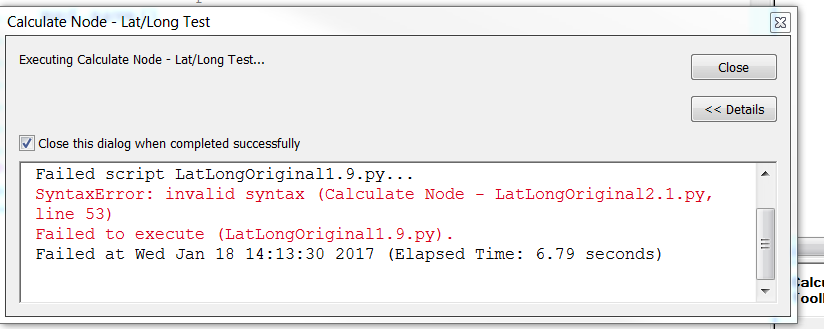# Conditional Statement with ArcPy.GetParameters as Text, Need to Update Latitude and Longitude Based on User Pref

1749
8
01-18-2017 10:17 AMNew Contributor

#python #ArcPy #for #syntaxerror

I am updating this tool that calculates the latitude and longitude values for all node poles. Originally the user could select Original Latitude and Longitude or Adjusted Latitude and Longitude.  The selection was for one or the other.  The update allows the user to select both.  It utilizes #arcpy.GetParametersAsText .  I created a new variable for the selection of Both, Original and Adjusted.  In doing this, I created a syntax error in my conditional statement ( line 49), and I cannot figure out how to fix it.  Any help on the error (or completing the tool, I imagine I have a few more errors left) would be much appreciated.

``````#########################################################################################################################
# Name: Calculate Node - Locations
# Author: Heather Wigell
# Description: This tool calculates the latitude and longitude values for all node poles
##################################################################
#########################
# Import system modules
#########################
import arcpy

#########################
# Set universal variables
#########################
nodeFeatures = r'OSP Mapping Layers\Node Locations'
inTable = nodeFeatures
mxd = map.MapDocument("CURRENT")
################
#parameters
arcpy.GetParameterAsText(0) = "Original or Adjusted Lat Long"
displayName = "Original or Adjusted Lat Long"
datatype = 'String'
direction = 'Input'
filters.type = 'ValueList'

######################
#local variables
latField = "OriginalLatitude"
longField = "OriginalLongitude"
mxd = map.MapDocument("CURRENT")
###########

#############
#Print Parameter Value (User Input {Original, Adjusted, Both}) on Line 49.

arcpy.GetParameterAsText(0)

arcpy.SetParameterAsText(0,x)

x

###############
#pick original, adjusted, or both and match it to ValueList
def(x)
if:
x == "Original" = 'true'
and x == "Original" = 'true'
latField = "OriginalLatitude"
longField = "OriginalLongitude";

elif x == "Adjusted" = 'true'
and x = "Adjusted" = 'true'

else: x == "Both" = 'true'
btlatField == "BothLatitude"
btlongField = "BothLongitude"

#########################
# Calulate latitude
#########################

# Start message
arcpy.AddMessage("Calculating the latitude values for all node features...")

# Set local variables
expression = "!Shape.Centroid.Y!"

# Execute CalculateField
arcpy.CalculateField_management(inTable, latField, adlatField, btlatField, expression, "PYTHON_9.3", "")

# End message
arcpy.AddMessage("The latitude values have been calculated for all node features!")

#########################
# Calculate Longitude
#########################
# Start message
arcpy.AddMessage("Calculating the longitude values for all node features...")

# Set local variables
expression = "!Shape.Centroid.X!"

# Execute CalculateField
arcpy.CalculateField_management(inTable, longField, adlongField, btlongField, expression, "PYTHON_9.3", "")

# End message
arcpy.AddMessage("The longitude values have been calculated for all node features!")

Tags (2)
8 RepliesOccasional Contributor III

Could you post a screenshot of the error? Your line 49 appears to be blank, so it's probably not causing the syntax error.Regular Contributor III
``arcpy.SetParameterAsText in this case the index number should be 1 you already have``arcpy.GetParameterAsText index set at 0. also you need to set x = "something" before set parameter as text``New Contributor

Originally, the error was on error Line 54. I added to the code and now I am getting an error on 53 also.  They are both generating the same errors.byMVP Esteemed Contributor

Since the Syntax Highlighter can do funky things with formatting at times, can you upload the script in a ZIP file?New Contributor

Joshua,

Thank you for your comments.  Here is the zip file.  The original project file has CP at the end.  You will see, I changed directions a few times.  Zip file

Regards,

HeatherbyMVP Regular Contributor

I think line 48 in the 2.1 version of the script in the zip file (lines 48-52 in the code block of your original post) should read:

``x = arcpy.GetParameterAsText(0)‍‍``

Also lines 53-67 of the zip file (lines 57-71 in your original post) has indentation problems.  This section does not appear to be a properly defined function, and the if else syntax appears incorrect.New Contributor

Thank you, Randy. 57-71 is what I was referring to in my question with syntax.  Do you have any specific advice on this section? Or anyone else?byMVP Regular Contributor

If I am understanding what you are trying to accomplish, I would suggest something like:

``````import arcpy

# set up  the input table

nodeFeatures = r'OSP Mapping Layers\Node Locations'
inTable = nodeFeatures

# GetParametersAsText will set latField and longField to the
# names of the field to be calculated

x = arcpy.GetParameterAsText(0)

if x == "Original":
latField = "OriginalLatitude"
longField = "OriginalLongitude"

else:
# assume x == "Both" (or add error code as needed)
latField = "BothLatitude"
longField = "BothLongitude"

# the calculation expression

expression = "!Shape.Centroid.Y!"

# CalculateField_management (in_table, field, expression, {expression_type}, {code_block})
# help page: http://desktop.arcgis.com/en/arcmap/10.3/tools/data-management-toolbox/calculate-field.htm

arcpy.CalculateField_management(inTable, latField, expression, "PYTHON_9.3")

expression = "!Shape.Centroid.X!"

arcpy.CalculateField_management(inTable, longField, expression, "PYTHON_9.3")
‍‍‍‍‍‍‍‍‍‍‍‍‍‍‍‍‍‍‍‍‍‍‍‍‍‍‍‍‍‍‍‍‍‍‍‍‍‍‍‍‍‍‍‍‍‍‍‍‍‍‍‍‍‍‍‍‍‍‍‍‍‍‍‍‍‍‍‍‍‍‍‍‍‍‍‍‍‍‍‍‍‍‍‍‍‍‍‍‍‍‍‍‍‍‍‍‍‍‍‍‍‍‍‍‍‍‍‍‍‍‍‍‍‍‍‍‍‍‍‍‍‍‍‍‍‍‍‍‍‍‍‍‍‍‍‍‍‍‍‍‍‍‍‍‍‍‍‍‍‍‍‍‍‍‍‍‍‍‍‍‍``````

Please note that I have simplified the code to show the major parts.  I may have omitted code that you are using for other purposes.